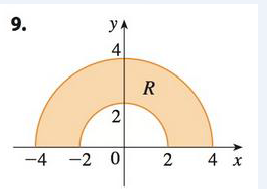Chapter 15, Problem 9RE

Chapter
Section
Textbook Problem

Write ∬ R f ( x ,   y )   d A as an iterated integral, where R is the region shown and f is an arbitrary continuous function on R.To determine

To write: The integral Rf(x,y)dA as an iterated integral.

Explanation

Calculation:

From the given figure, it is observed that the given portion is an annular region with two circles of radius 2 and 4. Since, the annular region is given, use of polar coordinates is more appropriate to use with. From the given figure, it is observed that r varies from 2 to 4 and θ varies from 0 to 2π . Substitute x=rcosθ,y=rsinθ . Thus, by the equation (1), the required integral is given by, Rf(x,y)dA=0π

Still sussing out bartleby?

Check out a sample textbook solution.

See a sample solution

The Solution to Your Study Problems

Bartleby provides explanations to thousands of textbook problems written by our experts, many with advanced degrees!

Get Started

Equations Solve the equation graphically. 140. x+4=x25

Precalculus: Mathematics for Calculus (Standalone Book)

In Exercises 99106, factor out the greatest common factor from each expression. 102. 3x2/3 2x1/3

Applied Calculus for the Managerial, Life, and Social Sciences: A Brief Approach

Using tan2 x = sec2 x − 1, ∫ tan3 x dx =

Study Guide for Stewart's Single Variable Calculus: Early Transcendentals, 8th

In Exercises 57-62, Use Venn diagram to illustrate each statement. (AB)c=AcBc

Finite Mathematics for the Managerial, Life, and Social Sciences